Measurement Worksheets

Measurement Worksheets for Practice

Here is a graphic preview for all of the measurement worksheets. You can select different oblects and reading scales to practice measuring and reading real world examples.These measurement worksheets are randomly created and will never repeat so you have an endless supply of quality measurement worksheets to use in the classroom or at home. Our measurement worksheets are free to download, easy to use, and very flexible.

These measurement worksheets are a great resource for children in Kindergarten, 1st Grade, 2nd Grade, 3rd Grade, 4th Grade, and 5th Grade.

Click here for a Detailed Description of all the Measurement Worksheets.

Quick Link for All Measurement Worksheets

Click the image to be taken to that Measurement Worksheet.

Reading a Tape MeasureMeasurement WorksheetsReading a Decimal RulerMeasurement WorksheetsReading a Metric RulerMeasurement Worksheets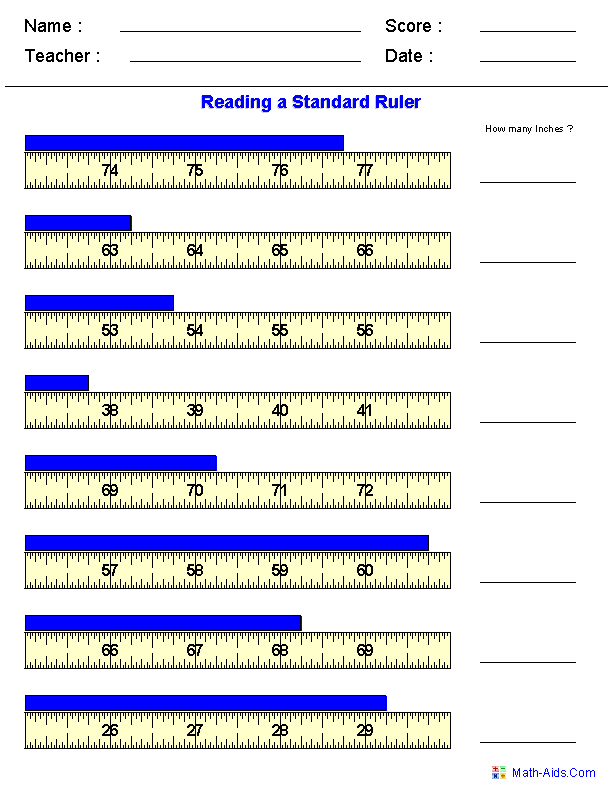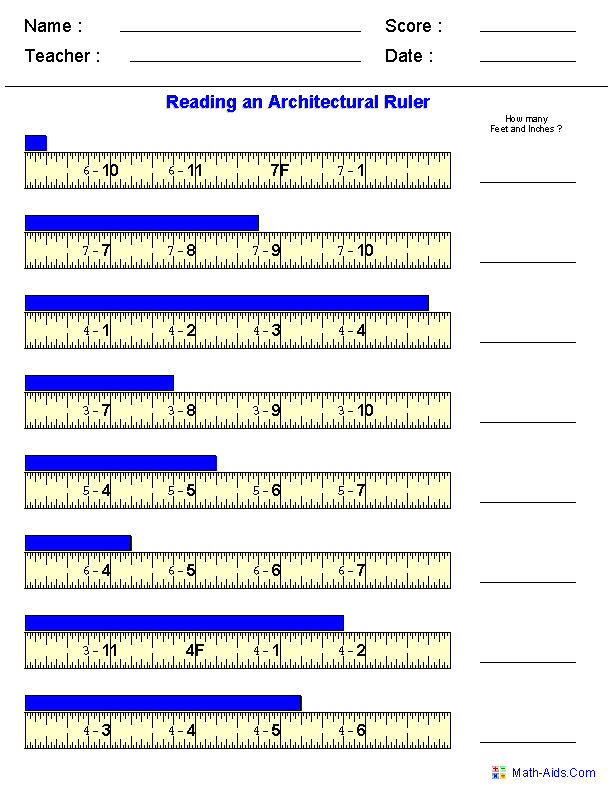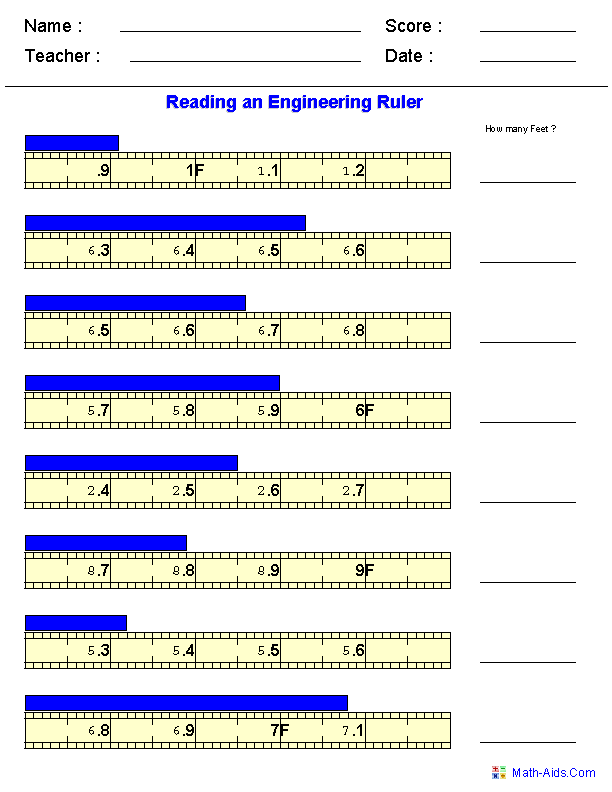Measuring In InchesMeasurement Worksheets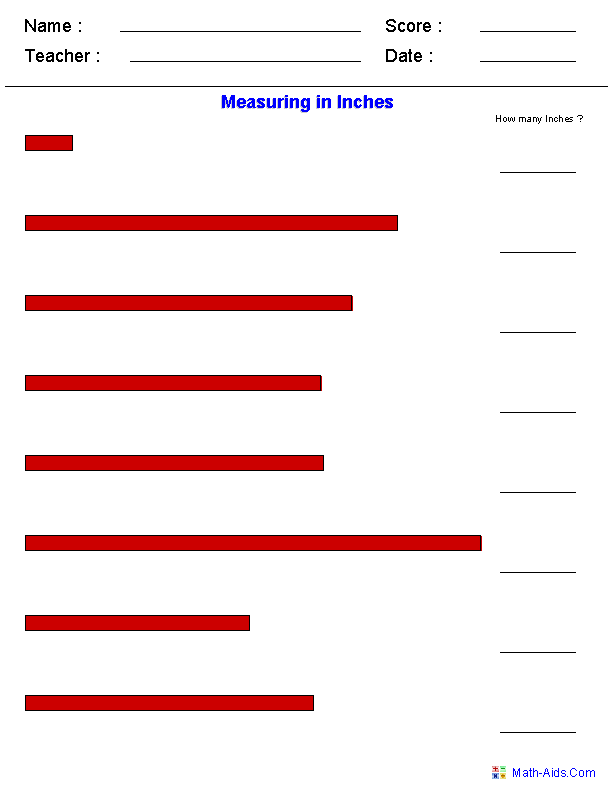Measuring In CentimetersMeasurement Worksheets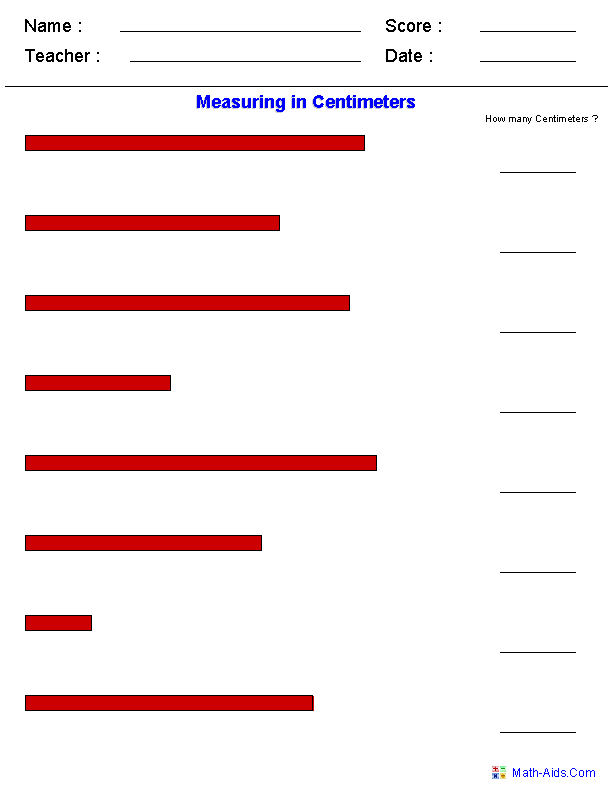Measuring Out LinesIn Inches or CentimetersAdding Irregular UnitsMeasurement WorksheetsSubtracting Irregular UnitsMeasurement Worksheets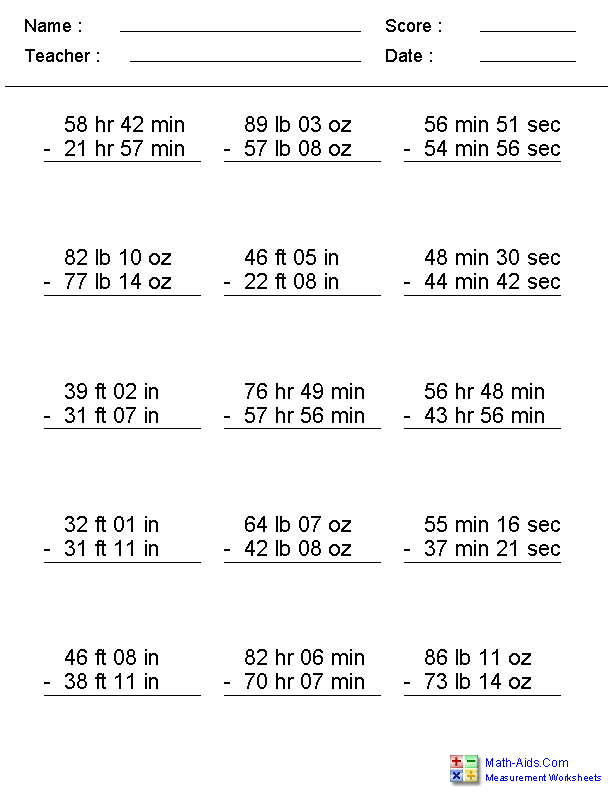Decimal Equivalents of Fractions for an InchMeasurement Worksheets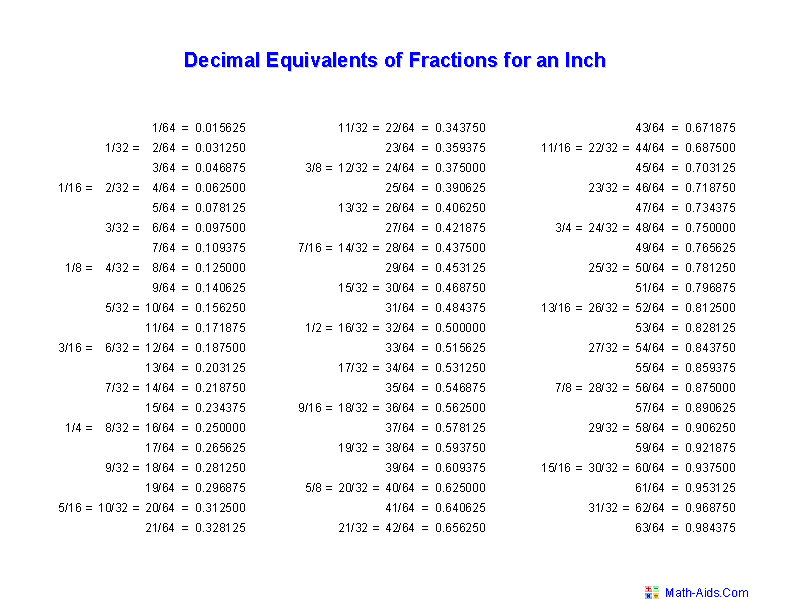Converting Feet & InchesMeasurement Worksheets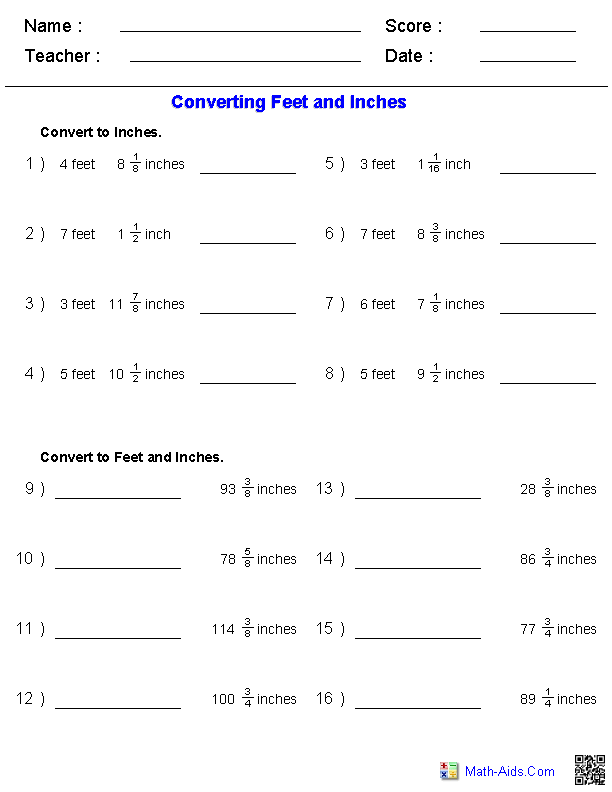Temperature ConversionsMeasurement WorksheetsFahrenheit & CelsiusMeasurement Worksheets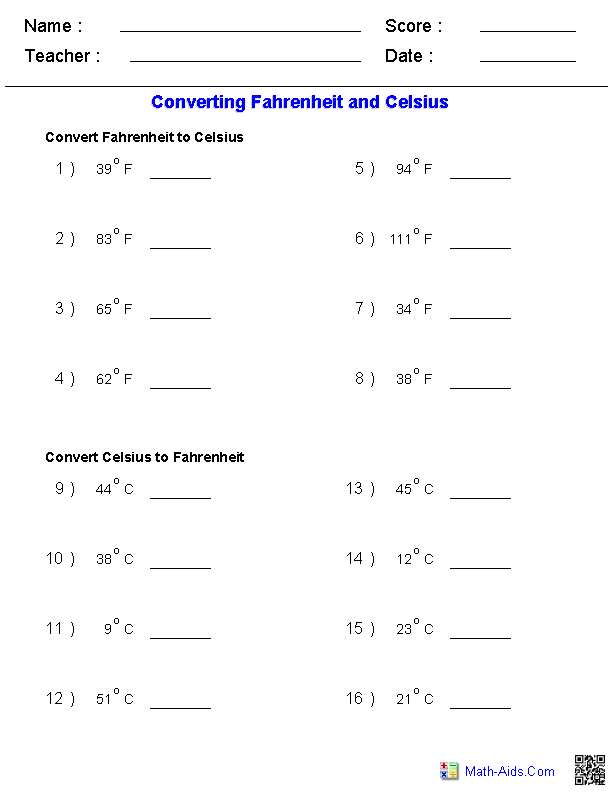Liquid Measure ConversionQuiz Worksheets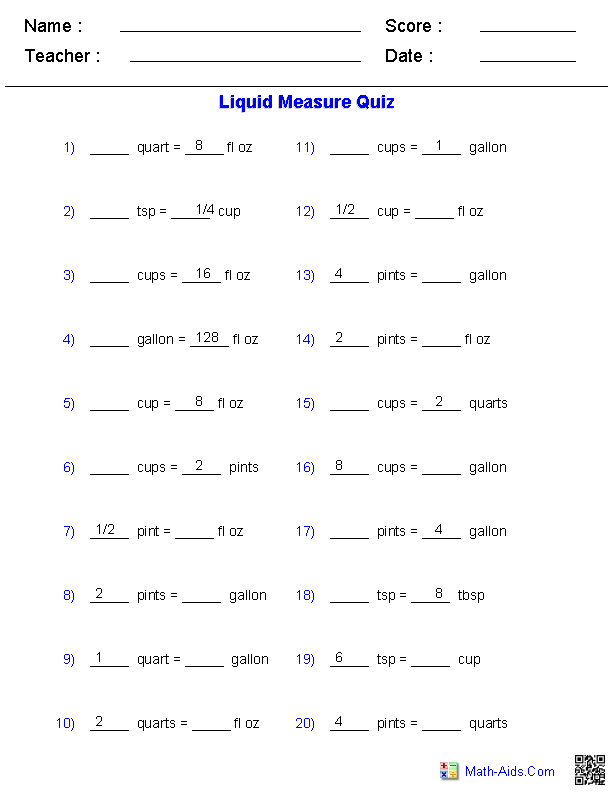Liquid Measure Table ConversionMeasurement Worksheets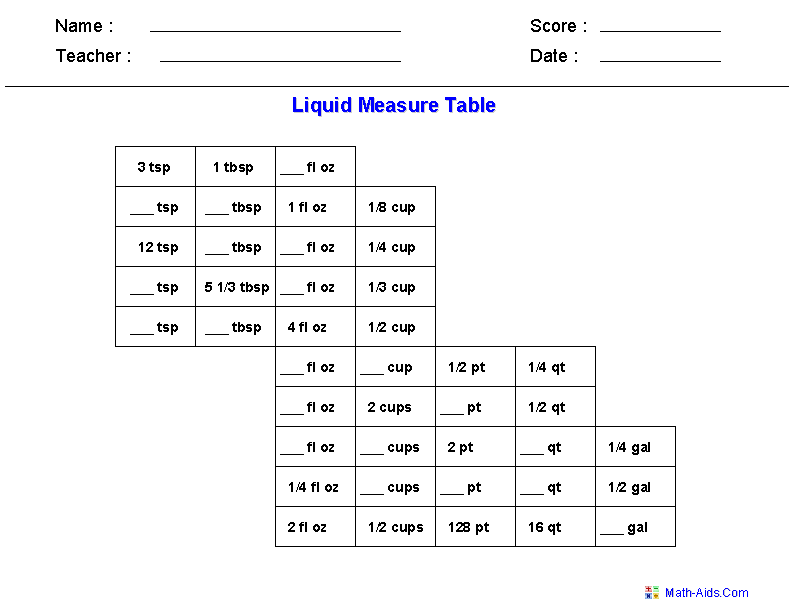General ConversionsMeasurement Worksheets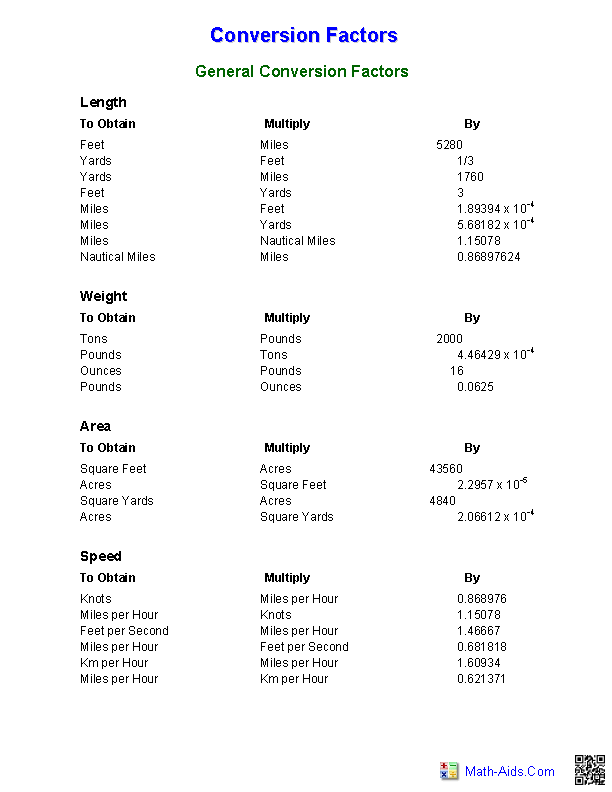General Conversion QuizMeasurement WorksheetsMetric ConversionQuiz Worksheets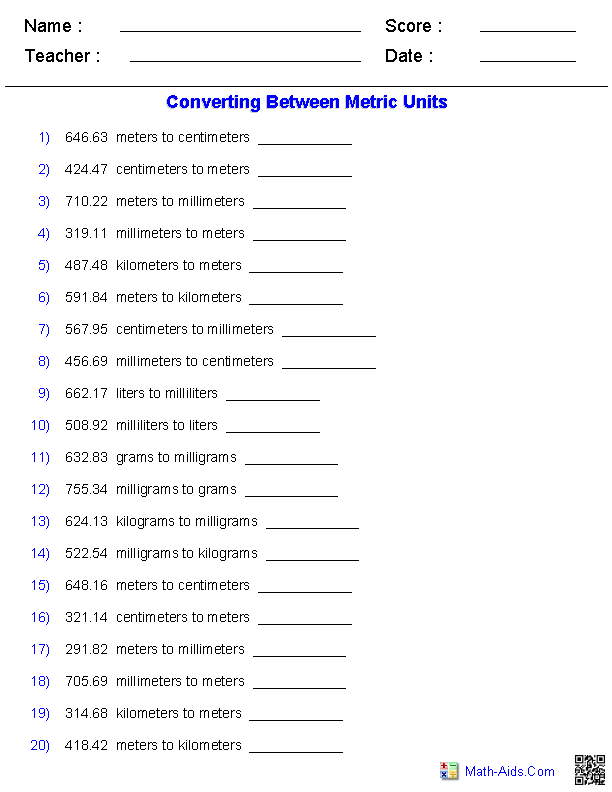English & Metric ConversionsMeasurement WorksheetsEnglish & Metric ConversionQuiz Worksheets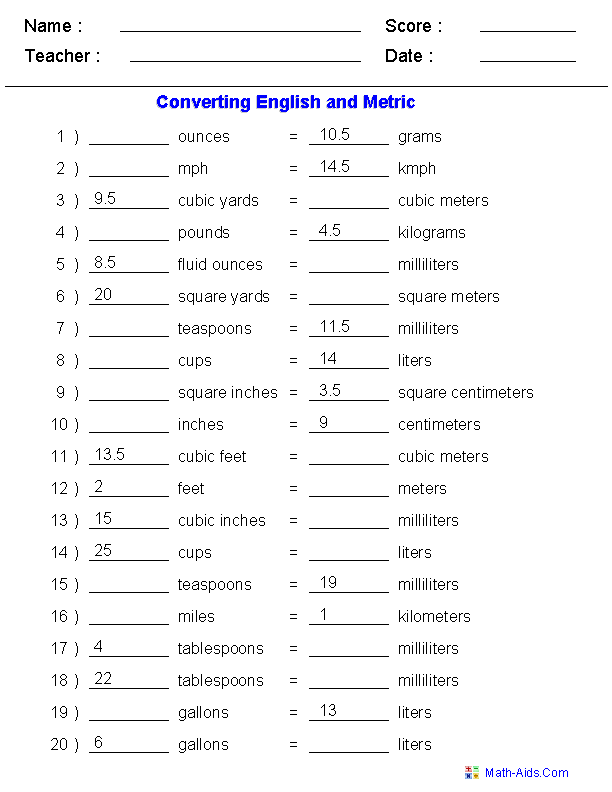Reading a Rain GaugeMeasurement Worksheets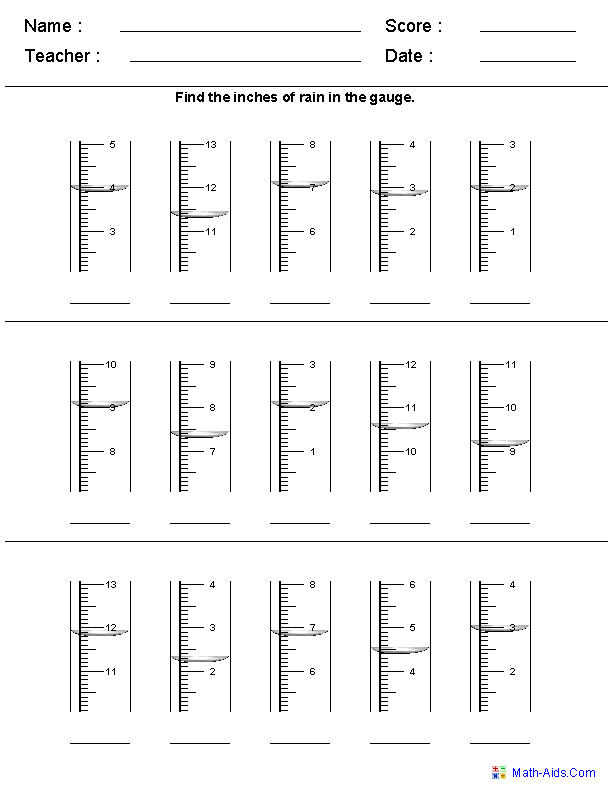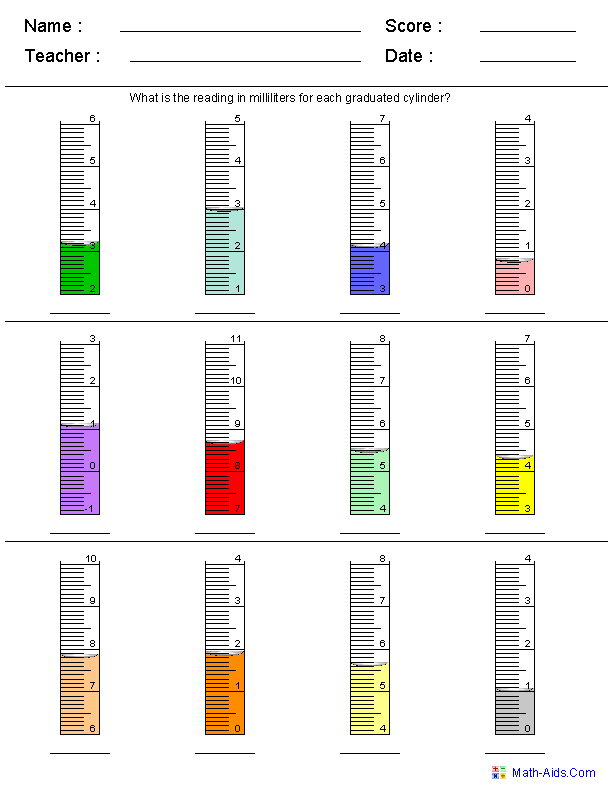Reading a ThermometerMeasurement Worksheets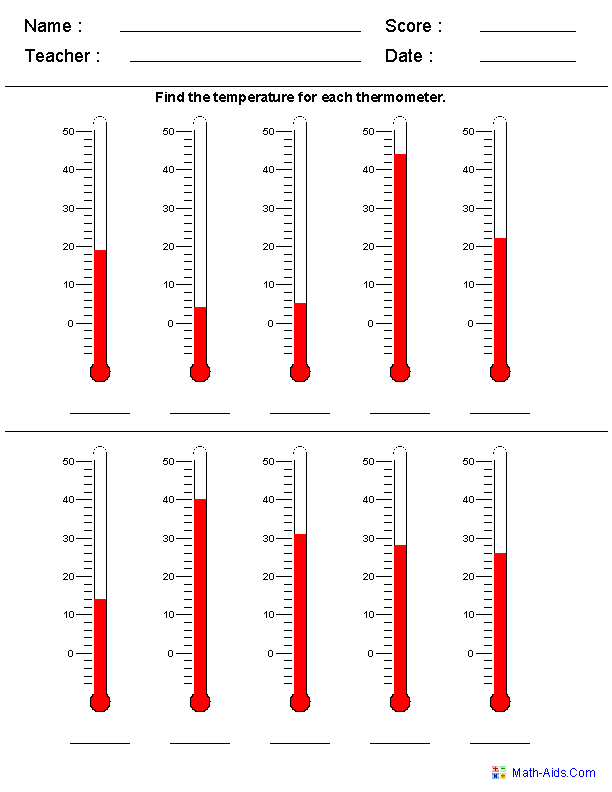Reading a BarometerMeasurement Worksheets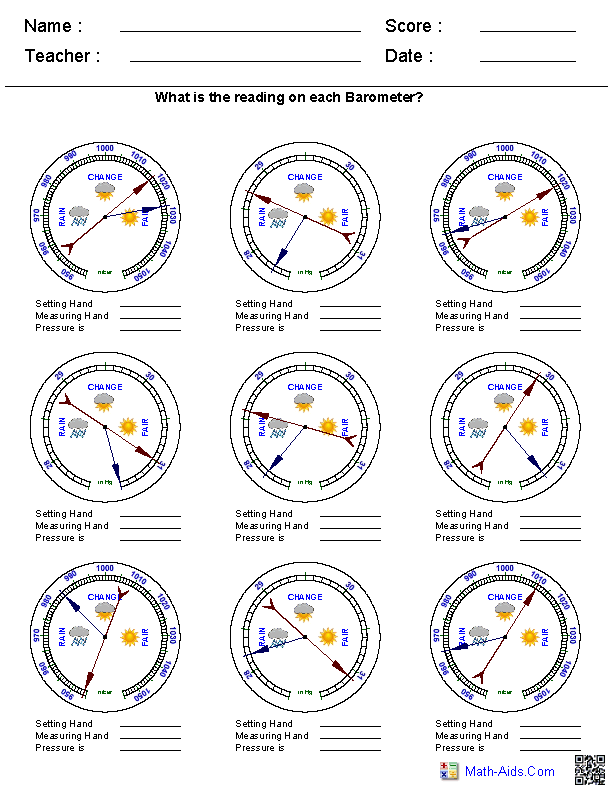Producing Protractor ImagesMeasurement Worksheets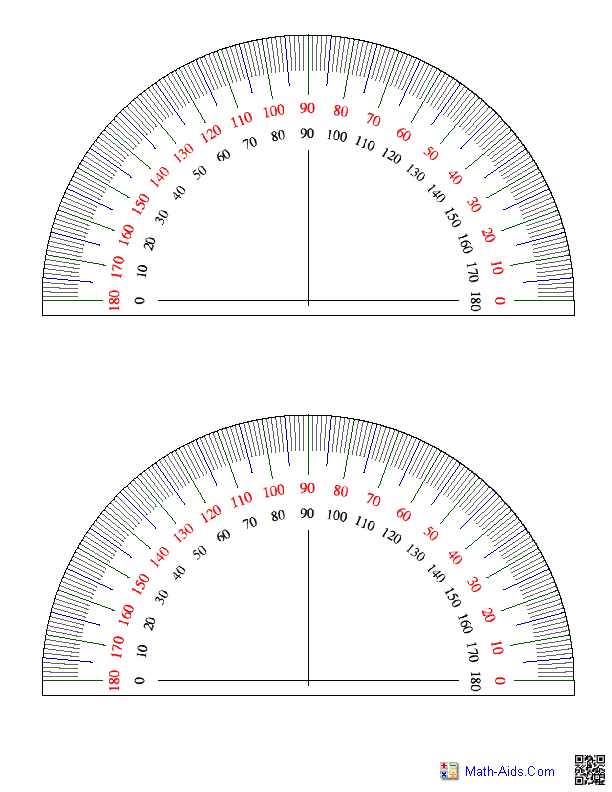Reading a ProtractorMeasurement Worksheets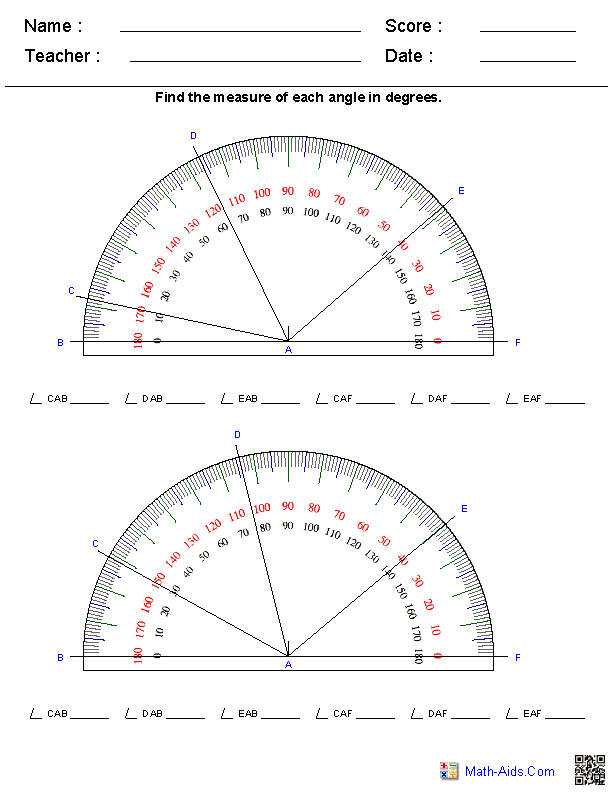Recommended Videos

Detailed Description for All Measurement Worksheets

Reading a Tape Measure Worksheets
These measurement worksheets are great for practicing reading a Tape Measure. These measurement worksheets will produce eight Tape Measure problems per worksheet.

Reading a Decimal Ruler Worksheets
These measurement worksheets are great for practicing reading a Decimal Ruler. These measurement worksheets will produce eight Decimal Ruler problems per worksheet.

Reading a Metric Ruler Worksheets
These measurement worksheets are great for practicing reading a Metric Ruler. These measurement worksheets will produce eight Metric Ruler problems per worksheet.

Reading Standard Measurements Worksheets
These measurement worksheets are great for practicing reading Standard Measurements. These measurement worksheets will produce eight Standard Measurement problems per worksheet.

Reading Architectural Measurements Worksheets
These measurement worksheets are great for practicing reading Architectural Measurements. These measurement worksheets will produce eight Architectural Measurement problems per worksheet.

Reading Engineering Measurements Worksheets
These measurement worksheets are great for practicing reading Engineering Measurements. These measurement worksheets will produce eight Engineering Measurements problems per worksheet.

Measuring In Inches Worksheets
These measurement worksheets are great for practicing measuring line segments in inches. These measurement worksheets will produce eight measuring problems per worksheet. You may choose to display the ruler or leave it off for the problems.

Measuring In Centimeters or Millimeters Worksheets
These measurement worksheets are great for practicing measuring line segments in centimeters. The measurement worksheet will produce eight measuring problems per worksheet. You may choose to display the ruler or leave it off for the problems. You then select if you want to measure centimeters or millimeters, and finally you select the increment to be used in the measuring problems.

Measuring Out Lines in Inches or Centimeters Worksheets
These measurement worksheets are great for practicing measuring out line segments in inches or centimeters. These measurement worksheets will produce ten measuring problems per worksheet.

Adding Irregular Units Worksheets
This measurement worksheet generator will produce problems to add irregular units of measurement. The problems may include Feet & Inches, Pounds & Ounces, Hours & Minutes, Minutes & Seconds, and Yard & Feet.

Subtracting Irregular Units Worksheets
This measurement worksheet generator will produce problems to subtract irregular units of measurement. The problems may include Feet & Inches, Pounds & Ounces, Hours & Minutes, Minutes & Seconds, and Yard & Feet.

Decimal Equivalents of Fractions for an Inch Worksheets
These measurement worksheets are great handouts for student learning about fractions and the decimal equivalents for an inch. They will produce a table of all the fractions from 1/64th to 63/64th of an inch.

Converting Feet & Inches Worksheets
These measurement worksheets are great for practicing converting feet and inches. These measurement worksheets will produce twenty conversion problems per worksheet. These measurement worksheets will use 1/2's, 1/4's, 1/8's. 1/16's and there is an option to select 1/32's and 1/64's.

Temperature Conversion Tables Worksheets
These measurement worksheets are great handouts for students containing temperature conversion factors.

Fahrenheit & Celsius Conversion Worksheets
These measurement worksheets are great for practicing converting between Fahrenheit and Celsius. These measurement worksheets will produce twenty conversion problems per worksheet.

Liquid Measure Conversion Quiz Worksheets
These measurement worksheets are great for practicing converting different liquid measure units. These measurement worksheets will produce twenty questions on different liquid measuring units per worksheet.

Liquid Measure Conversion Table Worksheets
These measurement worksheets are great for learning the different liquid measure units. These measurement worksheets will produce a table of equivalent liquid measuring units for the student to work with. You may select to leave some blank or completely fill out the table.

General Conversion Tables Worksheets
This measurement worksheet is a great handout for students containing general conversion factors for length, weight, area, and speed.

General Conversion Quiz Worksheets
This Measurement Worksheet is great for practicing converting length, weight, area, and speed. The measurement worksheet will produce sixteen conversion problems per worksheet.

Metric Conversion Quiz Worksheets
This measurement worksheets are great for practicing converting between different Metric units. These measurement worksheets will produce twenty conversion problems per worksheet.

English & Metric Conversion Tables Worksheets
These measurement worksheets are a great handout for the student containing a list of length, distance, area, volume, weight and speed conversions between English and Metric Units.

English & Metric Conversion Quiz Worksheets
This measurement worksheets are great for practicing converting between English and Metric units. These measurement worksheets will produce twenty conversion problems per worksheet.

Reading a Rain Gauge Worksheets
These measurement worksheets are great for practicing reading a Rain Gauge to measure rain fall. You may select inches or centimeters for the problems. These measurement worksheets will produce fifteen problems per worksheet.

These measurement worksheets are great for practicing reading graduated cylinders to measure liquid volume. You may select different types of cylinders for the problems. These measurement worksheets will produce twelve problems per worksheet.

Reading a Thermometer Worksheets
These measurement worksheets are great for practicing reading a Thermometer to measure temperatures. You may vary the scales for the problems and choose between Fahrenheit or Celcius. These measurement worksheets will produce ten problems per worksheet.

Reading a Barometer Worksheets
These measurement worksheets are great practicing reading a Barometer to measure barometric change. You may vary the scales for the problems. These measurement worksheets will produce nine problems per worksheet.

Producing Protractor Images Worksheets
These measurement worksheets are great for handouts, using on a smart board, or overhead projector where a protractor image is needed. These measurement worksheets will produce one, two, four, or six protractors per worksheet.

Reading a Protractor Worksheets
These measurement worksheets are great for practicing reading and using a protractor to measure angles. These measurement worksheets will produce two problems per worksheet.